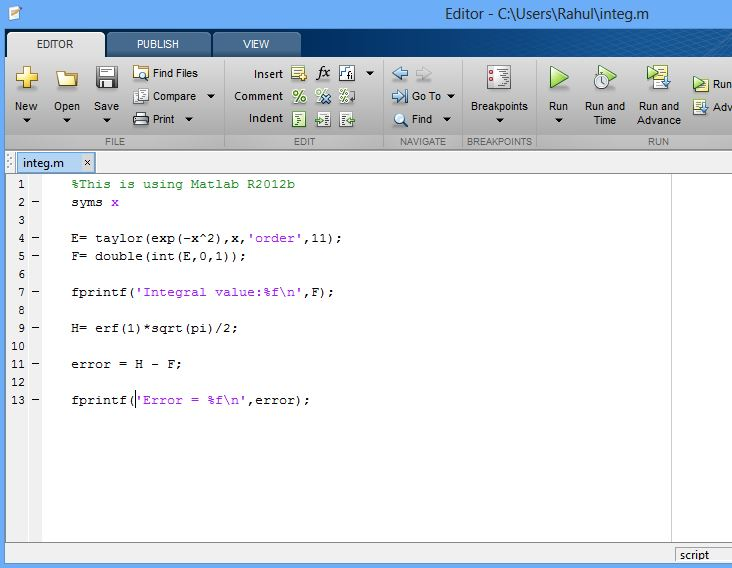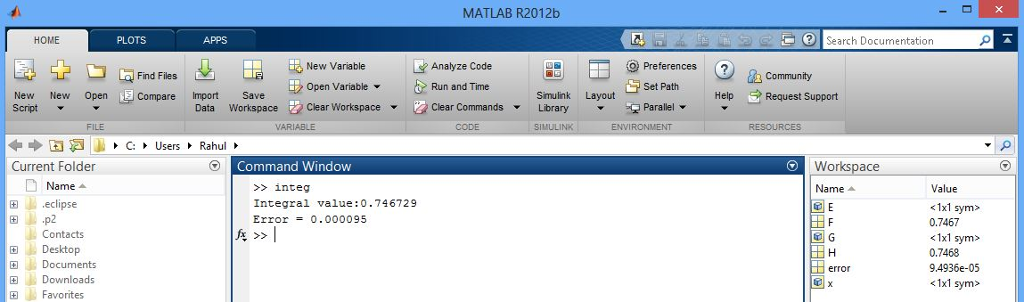# Answered! Matlab Question Integrals that cannot be evaluated in closed form sometimes can be evaluated approximately by using a series…

Matlab Question

Integrals that cannot be evaluated in closed form sometimes can be evaluated approximately by using a series representation for the integrand. For example, the following integral is used for some probability calculations:

Don't use plagiarized sources. Get Your Custom Essay on
Answered! Matlab Question Integrals that cannot be evaluated in closed form sometimes can be evaluated approximately by using a series…
GET AN ESSAY WRITTEN FOR YOU FROM AS LOW AS \$13/PAGE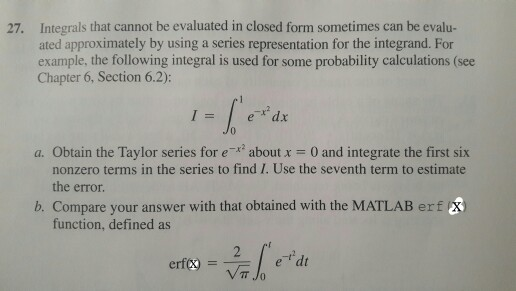I found a solution to this question, but I’m having trouble inputting it into matlab. Can someone explain it further for me (what suppose to be in the script file, the command window, what’s missing from the solution etc.), or if you have a different way to solve this question please do share.

The solution I found is:

(a)
syms x
E= taylor(exp(-x^2),11);
F= double(int(E,0,1)
F=
0.7467
G= taylor(exp(-x^2),13);
H= double(int(G,0,1))
H=
0.7468
error= H-F
error=
1.0684-004

(b)
erf(1)*sqrt(pi)/2
ans=
0.7468

The script file is given below. Save the script and run it. It should give the result

(A)

%This is using Matlab R2012b
syms x

E= taylor(exp(-x^2),x,’order’,11);
F= double(int(E,0,1));

fprintf(‘Integral value:%fn’,F);

G= taylor(exp(-x^2),x,’order’,13);
H= double(int(G,0,1));

error = H – F;

fprintf(‘Error = %fn’,error);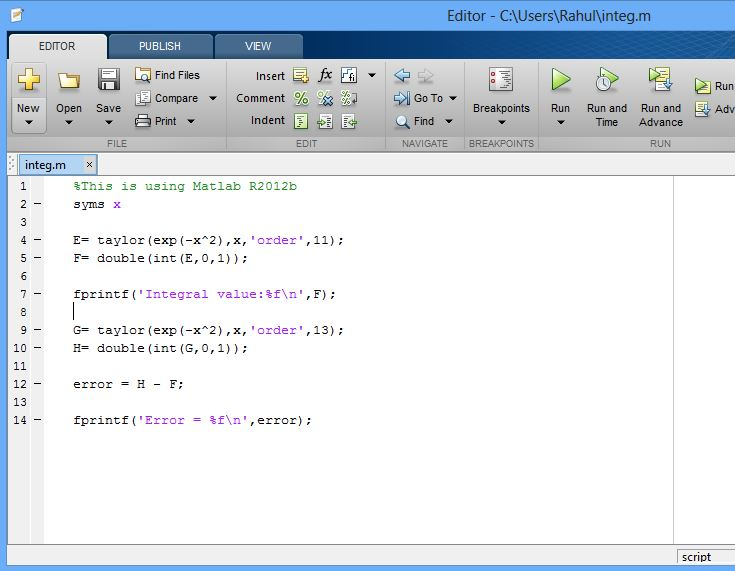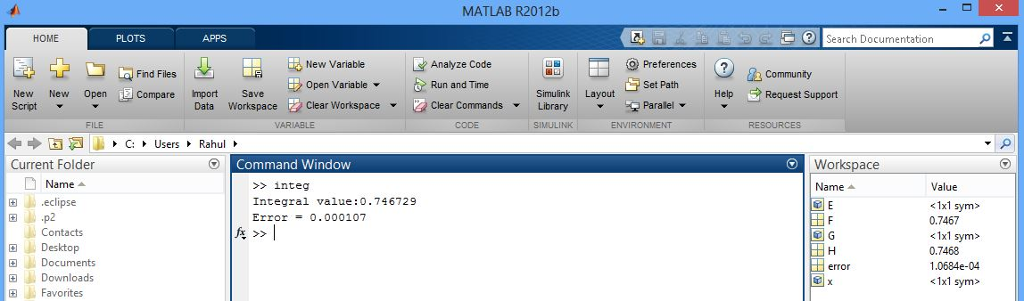(B)

%This is using Matlab R2012b
syms x

E= taylor(exp(-x^2),x,’order’,11);
F= double(int(E,0,1));

fprintf(‘Integral value:%fn’,F);

H= erf(1)*sqrt(pi)/2;

error = H – F;

fprintf(‘Error = %fn’,error);